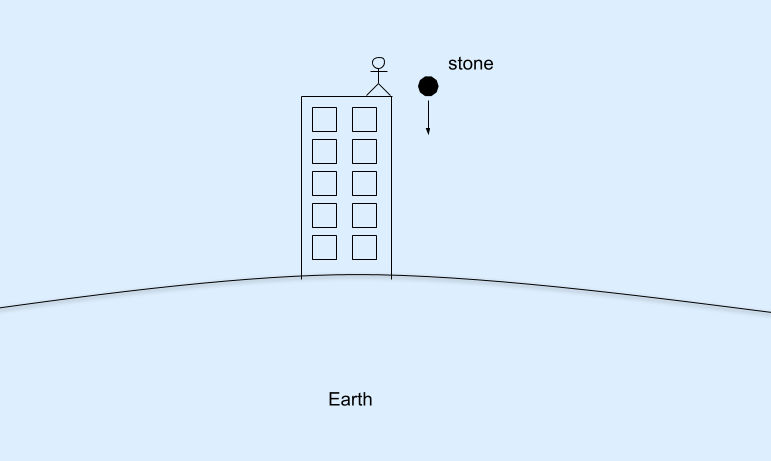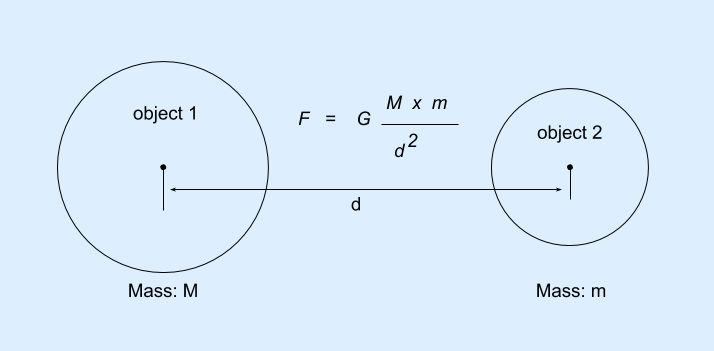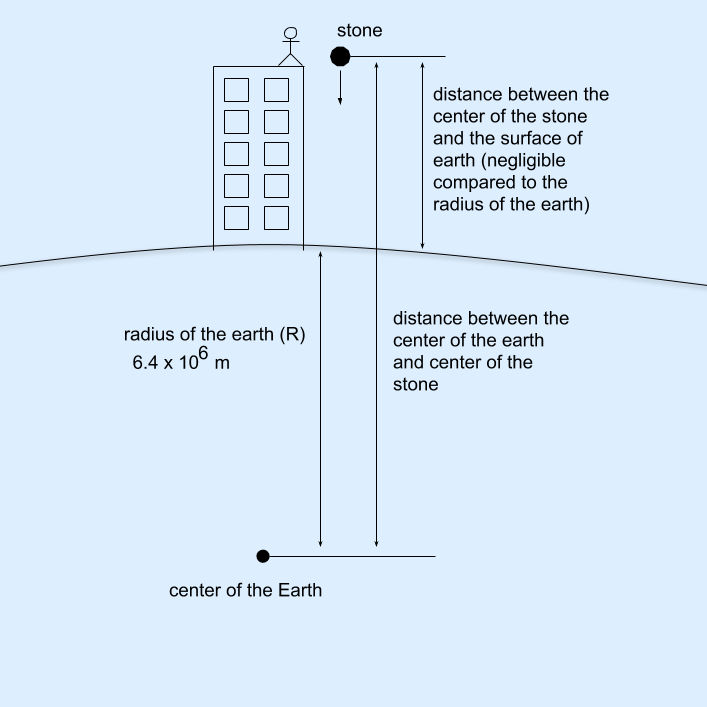# Why Gravitational Acceleration g is 9.8 m/s² ? [Calculation]

Earth and other planets attract objects towards itself with some force which depends on the mass and the distance between them. In most of the cases, we say that the object is being attracted towards the planet because of the size and the mass of the object is negligible compared to that of the planet. Since the planet is a huge and a heavy body, it will not get attracted or move towards the object, until and unless the object is another heavy body with a considerable mass. This phenomenon of attraction is governed by Universal Law of Gravitation.

Now, let us understand and calculate with how much acceleration will the object move towards Earth under the effect of the Earth's gravitational force.

## Example to understand the gravitational acceleration (g) of the Earth

A person standing on the top of a building drops a stone without applying any force as shown in the figure below. The stone will start moving in downward direction and will perform an accelerated motion towards the surface of the Earth.Person dropping stone from top of a building

This is because according to Newton's law of gravitation, the force of attraction between any two particles or objects is given by

$F = G \times {M \times m \over d^2} \quad ---- (1)$Gravitational force acting on two objects according to universal law of gravitation

Now according to the second law of motion, we know that the acceleration acting on any body is dependent on two things:

1. The total force acting on the object (in our example, only the gravitational force)
2. The mass of the object

Mathematically,

\begin{aligned} &F = m \times a \\ \\ &Where, \\ &F = \text{the force acting on the body} \\ &m = \text{the mass of the body} \\ &a = \text{acceleration with which the body the moving} \\ \end{aligned}

We know that, in our example the stone will move downwards with a force that the Earth attracts the stone with. And the acceleration will be the gravitational acceleration of the Earth, which we denote as g. Hence in the above equation, we can replace a the acceleration with g the gravitational acceleration. Which gives us:

$F = m \times g \quad ---- (2)$

In our example, this is the force that will act on the stone that was dropped from the building. And the variables will be

\begin{aligned} &M = \text{mass of the Earth} \\ &m = \text{mass of the stone} \\ &G = \text{gravitational constant} \\ &d = \text{the distance between the center of the stone and the center of the Earth} \end{aligned}The distance between stone and the surface of the Earth is negligible compared to the radius of Earth

From equation (1) and equation (2), we can say that:

\begin{aligned} m \times g &= G \times {M \times m \over d^2} \\ \cancel{m} \times g &= G \times {M \times \cancel{m} \over d^2} \\ g &= G \times {M \over d^2} \end{aligned}As mentioned earlier, d is the distance between the center of the stone and the center of the Earth. We can replace d with the radius of the Earth R because the distance from the center of the stone to the Earth is negligible compared to the radius of the Earth(as seen in the image above). Hence we get the equation:

$g = G \times {M \over R^2} \quad ---- (3)$

Now, to find the value of g, we just need to replace the values that we already know in the equation above.

## Find the value of gravitational acceleration - step by step calculation

Also watch detailed step-by-step explanation for derivation of value of $g= 9.8 \ m/s^2$ in our video.

Tip: The three values shown below are to be remembered along with the unit. This will also help you solve other numerical.

\begin{aligned} &M = \text{mass of the Earth} = 6 \times 10^{24} \thickspace kg \\ &G = \text{gravitational constant} = 6.7 \times 10^{-11} \thickspace N m^2 / kg^2 \\ &R = \text{radius of the Earth} = 6.4 \times 10^6 \thickspace m \end{aligned}

Putting all these values in equation (3):

\begin{aligned} g &= {6.7 \times 10^{-11} \times 6 \times 10^{24} \over (6.4 \times 10^6)^2} \\ \\ &= {6.7 \times 6 \times 10^{24-11} \over 6.4 \times 6.4 \times 10^{6} \times 10^{6}} \\ \\ &\text{removing decimals to simplify the equation} \\ &= {67 \times 6 \times 10^2 \times 10^{13} \over 64 \times 64 \times 10^1 \times 10^{12}} \\ \\ &= {67 \times \cancel{6} \times 10^2 \times 10^{13} \over \cancel{64} \times 64 \times 10^1 \times 10^{12}} \\ \\ &= {67 \times 3 \times 10^{2+13-1-12} \over 32 \times 64} \quad \text{(applying rule: \ } a^m \times a^n = a^{m+n} \text{\ and \ } {a^m \over a^n} = a^{m-n} ) \\ \\ &= {201 \times 10^{2} \over 2048} \\ \\ &= {20100 \over 2048} \\ \\ &= 9.8144 \\ \\ &\text{rounding the value} \\ &= 9.8 \quad m / s^2 \end{aligned}

## Deriving the unit of gravitational acceleration

Tip: This is a bonus section just for extra information.

How do we know that the unit of g is $m / s^2$?
To understand that let us replace the units shown here to equation (3) rather than the values.

\begin{aligned} g &= G \times {M \over R^2} \\ \\ &= {N \cancel{m^2} \over kg^{\cancel{2}}} \times {\cancel{kg} \over \cancel{m^2}} \\ \\ &= {N \over kg} \quad ---- (4) \\ \end{aligned}

We know that, N (newton) is a unit of force. And according to the second law of motion $F = ma$. That means the unit of force can also be written as $kg \sdot m / s^2$. Replacing this in equation (4):

\begin{aligned} &= {N \over kg} \\ \\ &= {kg \sdot m / s^2 \over kg} \\ \\ &= {\cancel{kg} \times m \over s^2 \times \cancel{kg}} \\ \\ &= {m \over s^2} \end{aligned}

Hence we can say that the unit of gravitational acceleration is $m / s^2$.

## Conclusion

Any object falling towards the Earth will move towards the Earth with an acceleration of $9.8 \space m / s^2$.

## Gravitational Acceleration of Earth

Any object that is within the range of Earth's gravitational field will get attracted towards the Earth with a force. This force is called the force of gravitation of Earth(gravity). The acceleration with which the object moves towards Earth due to gravity is called Gravitational Acceleration.

Gravitational Acceleration is denoted by g (small g) and its value is $\thickspace 9.8 \ m/s^2$.

### Can you answer this now ???

What will be the value of g (gravitational acceleration) on the moon?